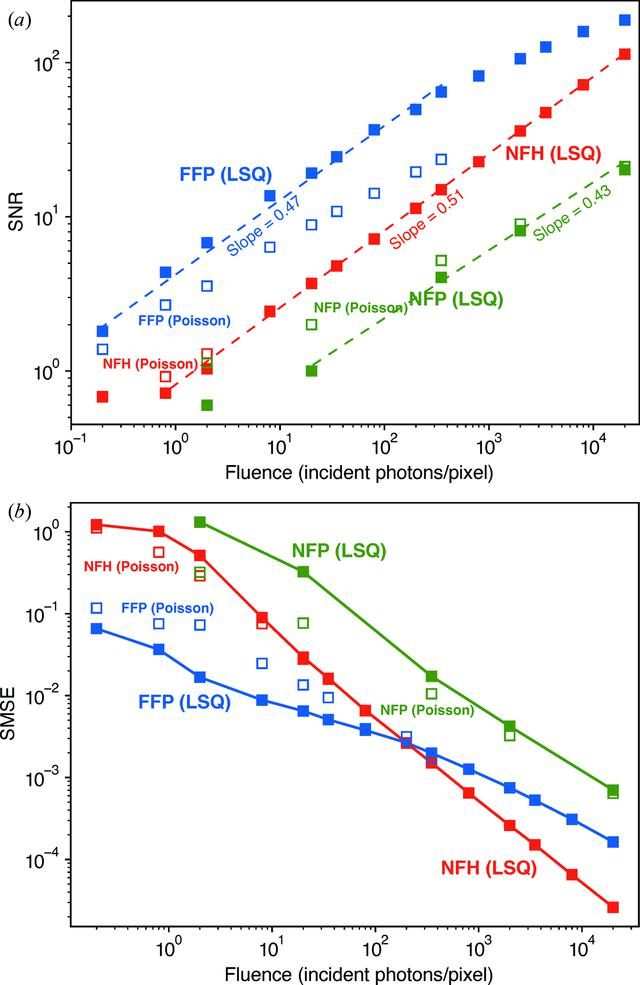disable zoom     view article Figure 3 Whole-image metrics of image-reconstructed image quality as a function of fluence nph. (a) The SNR as calculated using equation (14) for NFH, FFP and NFP, and using either the LSQ (solid squares) or Poisson cost function (open squares). The image correlation was calculated within the finite-support area of the object. At each photon fluence nph and for each cost function type, two separate instances of Poisson noise were generated and applied to the noise-free data set. The noisy data sets are then independently reconstructed and used for our correlation-based SNR calculation. The slope for the LSQ fitting curves is near 0.5 for all three techniques, indicating that the SNR increases roughly as (nph)1/2, as one might expect. (b) The within-support mean squared error (SMSE) of equation (15), which shows improved performance at low fluences for FFP compared with NFH. NFP shows a larger SMSE for all photon fluences tested.JOURNAL OFAPPLIEDCRYSTALLOGRAPHY
ISSN: 1600-5767
Volume 53| Part 3| June 2020| Pages 748-759